Anzeige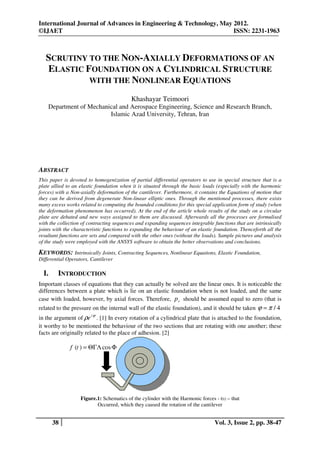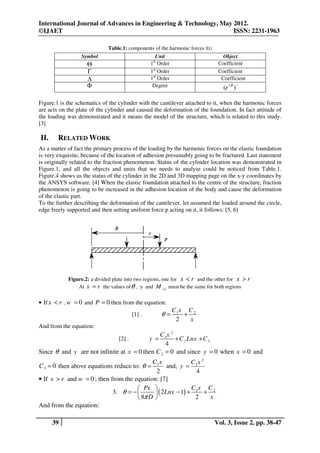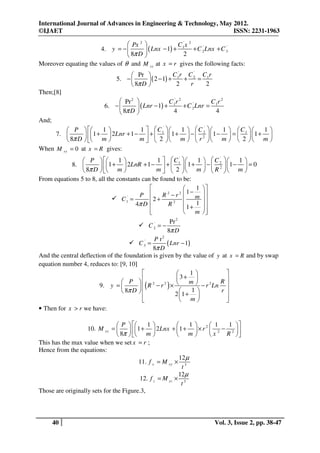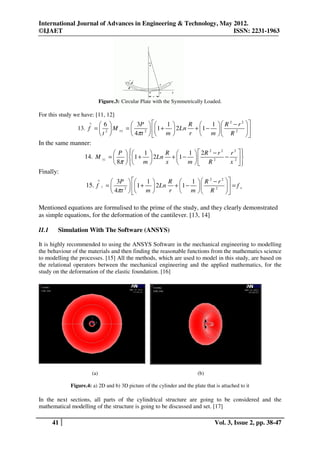Anzeige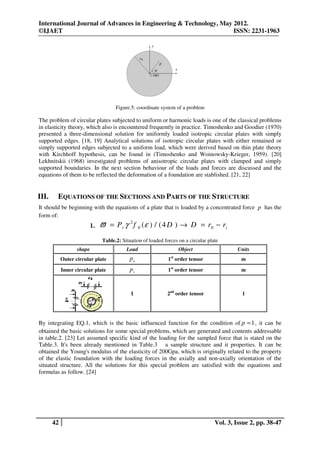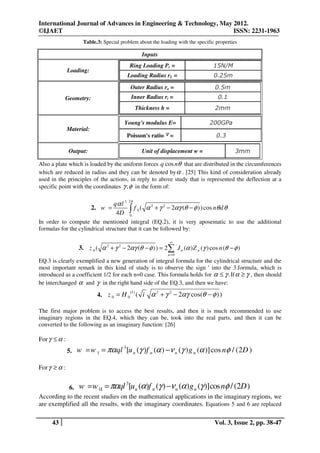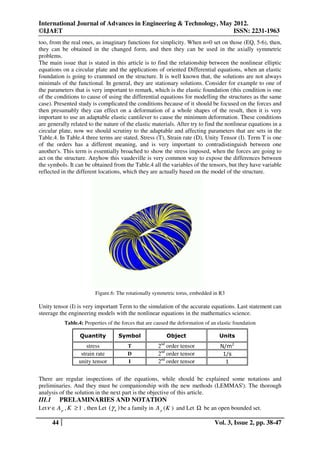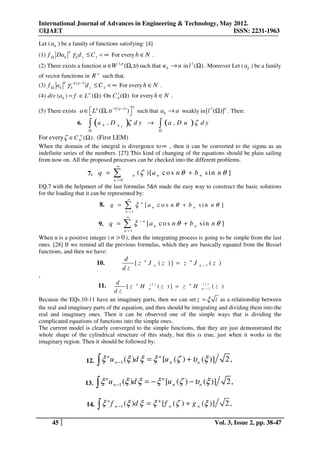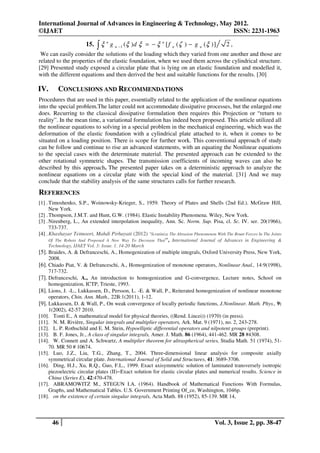Anzeige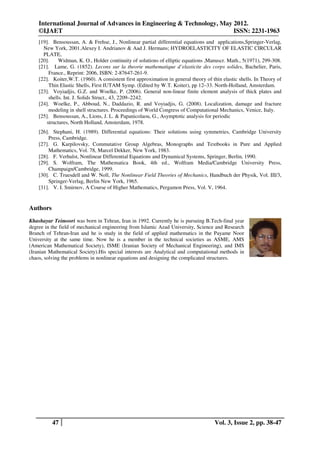Nächste SlideShareSolved exercises simple integration
1 von 10
Anzeige

### SCRUTINY TO THE NON-AXIALLY DEFORMATIONS OF AN ELASTIC FOUNDATION ON A CYLINDRICAL STRUCTURE WITH THE NONLINEAR EQUATIONS

1. International Journal of Advances in Engineering & Technology, May 2012. ©IJAET ISSN: 2231-1963 SCRUTINY TO THE NON-AXIALLY DEFORMATIONS OF AN ELASTIC FOUNDATION ON A CYLINDRICAL STRUCTURE WITH THE NONLINEAR EQUATIONS Khashayar Teimoori Department of Mechanical and Aerospace Engineering, Science and Research Branch, Islamic Azad University, Tehran, Iran ABSTRACT This paper is devoted to homogenization of partial differential operators to use in special structure that is a plate allied to an elastic foundation when it is situated through the basic loads (especially with the harmonic forces) with a Non-axially deformation of the cantilever. Furthermore, it contains the Equations of motion that they can be derived from degenerate Non-linear elliptic ones. Through the mentioned processes, there exists many excess works related to computing the bounded conditions for this special application form of study (when the deformation phenomenon has occurred). At the end of the article whole results of the study on a circular plate are debated and new ways assigned to them are discussed. Afterwards all the processes are formulised with the collection of contracting sequences and expanding sequences integrable functions that are intrinsically joints with the characteristic functions to expanding the behaviour of an elastic foundation. Thenceforth all the resultant functions are sets and compared with the other ones (without the loads). Sample pictures and analysis of the study were employed with the ANSYS software to obtain the better observations and conclusions. KEYWORDS: Intrinsically Joints, Contracting Sequences, Nonlinear Equations, Elastic Foundation, Differential Operators, Cantilever I. INTRODUCTION Important classes of equations that they can actually be solved are the linear ones. It is noticeable the differences between a plate which is lie on an elastic foundation when is not loaded, and the same case with loaded, however, by axial forces. Therefore, p r should be assumed equal to zero (that is related to the pressure on the internal wall of the elastic foundation), and it should be taken ϕ = π / 4 in the argument of ρe i ϕ .  In every rotation of a cylindrical plate that is attached to the foundation, it worthy to be mentioned the behaviour of the two sections that are rotating with one another; these facts are originally related to the place of adhesion.  f (t ) = ΘΓΛ cos Φ Figure.1: Schematics of the cylinder with the Harmonic forces - f(t) – that Occurred, which they caused the rotation of the cantilever 38 Vol. 3, Issue 2, pp. 38-47
2. International Journal of Advances in Engineering & Technology, May 2012. ©IJAET ISSN: 2231-1963 Table.1: components of the harmonic forces f(t) Symbol Unit Object st Θ 1 Order Coefficient Γ 1st Order Coefficient Λ 1st Order Coefficient Φ Degree (e i θ )o Figure.1 is the schematics of the cylinder with the cantilever attached to it, when the harmonic forces are acts on the plate of the cylinder and caused the deformation of the foundation. In fact attitude of the loading was demonstrated and it means the model of the structure, which is related to this study.  II. RELATED WORK As a matter of fact the primary process of the loading by the harmonic forces on the elastic foundation is very exquisite, because of the location of adhesion presumably going to be fractured. Last statement is originally related to the fraction phenomenon. Status of the cylinder location was demonstrated in Figure.1, and all the objects and units that we needs to analyse could be noticed from Table.1. Figure.4 shows us the status of the cylinder in the 2D and 3D mapping page on the x-y coordinates by the ANSYS software.  When the elastic foundation attached to the centre of the structure, fraction phenomenon is going to be increased in the adhesion location of the body and cause the deformation of the elastic part. To the further describing the deformation of the cantilever, let assumed the loaded around the circle, edge freely supported and then setting uniform force p acting on it, it follows: [5, 6] Figure.2: a divided plate into two regions, one for x < r and the other for x > r At x = r the values of θ , y and M xy must be the same for both regions • If x < r , w = 0 and P = 0 then from the equation: C 1x C 2  . θ= + 2 x And from the equation: C 1x 2  . y =+ C 2 Lnx + C 3 4 Since θ and y are not infinite at x = 0 then C 2 = 0 and since y = 0 when x = 0 and C 1x C 1x 2 C 3 = 0 then above equations reduce to: θ = and, y = 2 4 • If x > r and w = 0 ; then from the equation:   Px  C 1' x C 2' 3. θ = −   ( 2Lnx − 1) + +  8π D  2 x And from the equation: 39 Vol. 3, Issue 2, pp. 38-47
3. International Journal of Advances in Engineering & Technology, May 2012. ©IJAET ISSN: 2231-1963  Px 2  C 'x 2 4. y = −   ( Lnx − 1) + 1 + C 2' Lnx + C 3'  8π D  2 Moreover equating the values of θ and M xy at x = r gives the following facts:  Pr  C 'r C ' C r 5. −   ( 2 − 1) + 1 + 2 = 1  8π D  2 r 2 Then;  Pr 2  C 1' r 2 C 1r 2  ( Lnr − 1) + ' 6. −  + C 2 Lnr =  8π D  4 4 And;  P   1 1   C 1'   1   C 2'   1   C1   1 7.    1 +  2Lnr + 1 −  +   1 +  −  2  1 −  =   1 +   8π D   m  m   2  m   r  m   2  m  When M xy = 0 at x = R gives:  P   1 1  C '  1   C '  1  8.    1 +  2LnR + 1 −  +  1  1 +  −  2  1 − 2 =0  8π D   m  m   2  m   R  m  From equations 5 to 8, all the constants can be found to be:   1  1 −  P  R2 −r2  m C 1' = 2 +   4π D  R 2  1 + 1     m  Pr 2 C 2' = − 8π D P r2 C 3' = ( Lnr − 1) 8π D And the central deflection of the foundation is given by the value of y at x = R and by swap equation number 4, reduces to: [9, 10]   1   3+  R  P   R2 −r2 ×  m 9. y =    8π D   (  ) 1 − r 2 Ln  r 2 1 +     m   • Then for x > r we have:  P   1   1  2 1 1  10. M xy =   1 +  2Lnx + 1 +  × r  2 − 2   8π   m   m  x R  This has the max value when we set x = r ; Hence from the equations: 12 µ 11. f x = M xy × t3 12µ 12. f z = M yz × 3 t Those are originally sets for the Figure.3, 40 Vol. 3, Issue 2, pp. 38-47
4. International Journal of Advances in Engineering & Technology, May 2012. ©IJAET ISSN: 2231-1963 Figure.3: Circular Plate with the Symmetrically Loaded. For this study we have: [11, 12] ∧ 6   3P   1 R  1   R 2 − r 2  13. f =  2  M xy =  2   1 +  2Ln +  1 −    t   4π t   m  r  m   R 2  In the same manner: P     R    2R − r r 2   2 2 1 1 14. M yz =    1 +  2Ln + 1 −  2 − 2   8π   m   x  m  R x   Finally:  3P   1 R    R − r  ∧ 2 2 1 15. f z = 2   1 +  2Ln +  1 −  2  = f x  4π t   m  r  m  R  Mentioned equations are formulised to the prime of the study, and they clearly demonstrated as simple equations, for the deformation of the cantilever. [13, 14] II.1 Simulation With The Software (ANSYS) It is highly recommended to using the ANSYS Software in the mechanical engineering to modelling the behaviour of the materials and then finding the reasonable functions from the mathematics science to modelling the processes.  All the methods, which are used to model in this study, are based on the relational operators between the mechanical engineering and the applied mathematics, for the study on the deformation of the elastic foundation.  (a) (b) Figure.4: a) 2D and b) 3D picture of the cylinder and the plate that is attached to it In the next sections, all parts of the cylindrical structure are going to be considered and the mathematical modelling of the structure is going to be discussed and set.  41 Vol. 3, Issue 2, pp. 38-47